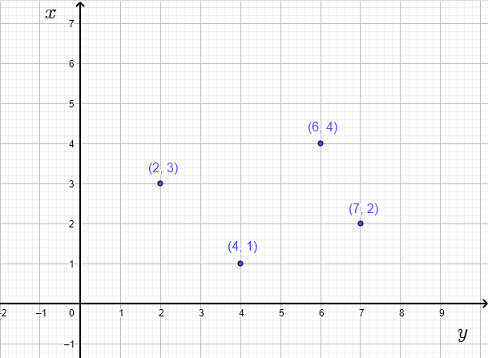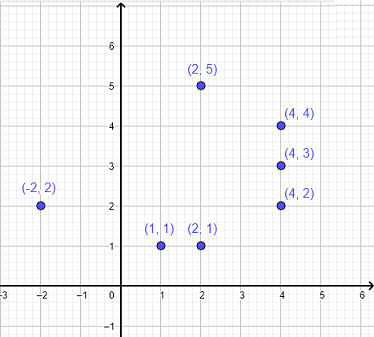# Functions in Mathematics

  

The definition of function in mathematics is presented along with examples, questions and their solutions.

## Definition of a Function in Mathematics

A function from a set $D$ to a set $R$ is a relation that assigns to each element $x$ in $D$ exactly one element $y$ of $R$. The set $D$ is the domain (inputs) and the set $R$ is the range (outputs) [1 2].

### Functions represented by Venn diagrams

Figures 1 and 2 below show Venn diagrams representing functions from $D$ to $R$ because to each input in $D$ corresponds one output only in $R$.
Note that two different inputs such as $3$ and $5$ having the same output $0$ do not contradict the definition of a function since each input has exactly one output.

Venn diagram in figure 3 is not a function because to the input $6$ in the domain $D$ corresponds two outputs $0$ and $-2$.

### Functions represented by ordered pairs

Which of the following relations represents a function?
a) $R_1 = \{ \; (a,2) \; , \; (b,4) \; , \; (c,7) \; , \; (d,-2) \}$
b) $R_2 = \{ \; (a,4) \; , \; (a,5) \; , \; (b,5) \; , \; (c,5) \}$
c) $R_3 = \{ \; (0,4) \; , \; (2,4) \; , \; (4,5) \; , \; (6,5) \}$
Solution
a) All elements of the domain of relation $R_1$ are different and therefore $R_1$
is a function .
b) To the input $a$ in the domain of $R_2$ corresponds two different outputs $4$ and $5$ and therefore according to the definition above, relation $R_2$ is
not a function .
c) All elements of the domain of relation $R_3$ are different and therefore $R_3$
is a function .

### Functions represented by graphs

Explain why the relations in figures 4 and 6 are not functions and the relation in figure 5 is a function?
a) The graph in figure 4 is
not a function because to the input $x = 2$ ( $x$coordinate ) corresponds two different outputs $4$ and $6$ ( $y$coordinate )Fig.4 - Relation Given by a Graph that is not a Function
b) The graph in figure 5 is
a function because to each input $x$ ( $x$coordinate ) corresponds exactly one output $y$ ( $y$coordinate ).Fig.5 - Relation Given by a Graph that is a Function
c) The graph in figure 6 is
not a function because to the input $x = 2$ corresponds two different outputs $1$ and $5$ and to the input $x = 4$ corresponds three different outputs $2, 3$ and $4$.Fig.6 - Relation Given by a Graph that is not a Function

### Functions Represented by Equations

Functions may be represented by mathematical equations as follows:
a)   $y = 2 x - 1$   ,   b)   $y = x^2 + 1$   ,   c)   $y = \dfrac {1}{x}$
All the above equations represent $y$ as function of $x$ because for each for each value of $x$ in the domain, we obtain exactly one value of $y$.
$x$ is called the independent variable and $y$ is the dependent variable.
More on functions represented by equations are included.

## Domain and Range of a Function

The domain $D$ of a function is the set of all input values for which the output is defined.
The range $R$ of a function is the set of all output values corresponding to the input values in the domain $D$.
Example
Find the domain and range of the functions defined in different forms:
a) A Set of ordered pairs: $\quad F_1 = \{ \; (a,2) \; , \; (b,4) \; , \; (c,7) \; , \; (d,-2) \}$
b) Venn diagrams

c) A graph
d) An equation: $\quad y = - x + 3$ for $x$ in the domain $D = \{-3,0,6,7\}$
Solution to Example
a)
The domain $D$ is the set of all inputs represented by the first elements in all ordered pairs. Hence $D = \{ a ,b, c , d \}$
The range $R$ is the set of all outputs corresponding to each element in the domain $D$. Hence; $R = \{ 2 ,4, 7 , -2 \}$
b)
The domain $D$ is the set of all inputs. Hence $D = \{ 0 ,5, 3 , -3 \}$
The range $R$ is the set of all outputs corresponding to each element in the domain $D$. Hence; $R = \{ 2 , 0 , 6 \}$
c)
The domain $D$ is the set of all inputs represented by the $x$ coordinates of the points of the graph representing the function. Hence $D = \{ 2 , 4 , 6 , 7 \}$
The range $R$ is the set of all outputs corresponding to each element in the domain $D$ and represented by the $y$ coordinates. Hence; $R = \{ 3 , 1 , 4 , 2 \}$
d) The domain is given explicitly as: $D = \{-3,0,6,7\}$
The range $R$ is the set of the values of $y$ calculated by substituting the values of $x$ in the equation of the function.
for $x = -3$ , $\quad y = - x + 3 = - (-3) + 3 = 6$
for $x = 0$ , $\quad y = - x + 3 = - (0) + 3 = 3$
for $x = 6$ , $\quad y = - x + 3 = - (6) + 3 = - 3$
for $x = 7$ , $\quad y = - x + 3 = - (7) + 3 = - 4$
Hence $R = \{ 6 , 3 , -3 , -4 \}$

## Questions

( solutions )

### Part A

Which of the following relations given by Venn diagrams is or is not a function? Explain why.

### Part B

Which of the following relations given by graphs is or is not a function? Explain why.

### Part C

Which of the following relations given by ordered pairs is or is not a function? Explain why.
a) $R_1 = \{ \; (-2,2) \; , \; (0,4) \; , \; (9,7) \; , \; (12,-2) \}$
b) $R_2 = \{ \; (a,0) \; , \; (a,7) \; , \; (a,9) \; , \; (a,5) \}$
c) $R_3 = \{ \; (0,4) \; , \; (2,4) \; , \; (5,4) \; , \; (9,4) \}$
d) $R_4 = \{ \; (a,b) \; , \; (b,a) \; , \; (c,e) \; , \; (d,a) \}$ , assuming that all letters in the ordered pairs have different values.

### Part D

The equation $y = x - 2$ represents $y$ as a function of $x$. Represent the function as
a) a table,
b) a set of ordered pairs,
c) a graph
d) a Venn diagram
for the values of $x$ in the domain: $D = \{ - 1 , 0 , 1 , 2\}$

### Part E

Find the domain and range of the functions represented by
a)
Venn diagrams

b)
the equation $y = \dfrac{1}{x+2}$ for the values of $x$ in the domain $D = \{ 0 , 1 , 4 \}$
c)
by the graph

## Solutions to the Above Questions

### Part A

a) All inputs in the domain of the relation in part a) has one corresponding output and therefore the relation in a) is a function .
b) The input $3$ of the relation in part b) has two corresponding outputs $A$ and $B$ and therefore the relation in b)
is NOT a function .
c) All inputs of the relation in part c) has one corresponding output and therefore the relation in a)
is a function .
d) The input $5$ of the relation in part d) has three corresponding outputs $a$, $b$ and $c$ and therefore the relation in b)
is NOT a function .

### Part B

a) The graph in part a) is a function because to each input ( $x$ coordinate ) corresponds one output ( $y$ coordinate ).
b) The graph in part b) is NOT a function because to the input $x = 1$ corresponds $2$ outputs: $y = 2$ and $y = - 2$.
c) The graph in part c) is NOT a function because to the input $x = 1$ corresponds $4$ outputs: $y = -3$, $y = - 1$, $y = 1$ and $y = 3$.
d) he graph in part d) is a function because to each input ( $x$ coordinate ) corresponds one output ( $y$ coordinate).

### Part C

a) All inputs, the first elements in the ordered pairs, are different and therefore $R_1$ is a function.
b) The input $a$ has more than one corresponding output value and therefore $R_2$ is NOT a function.
c) All inputs, the first elements in the ordered pairs, are different and therefore $R_3$ is a function.
d) All inputs are different and therefore $R_4$ is a function.

### Part D

Given the function $y = x - 2$ and its domain: $D = \{ - 1 , 0 , 1 , 2\}$,
a)
calculate the corresponding values of $y$ for the given values of $x$ and put them in a table.
For $\color{red} {x = - 1}$ , $\quad y = \color{red}x - 2 = \color{red}{-1} - 2 = -3$
For $\color{red} {x = 0}$ , $\quad y = \color{red}x - 2 = \color{red}0 - 2 = -2$
For $\color{red} {x = 1}$ , $\quad y = \color{red}x - 2 = \color{red}1 - 2 = -1$
For $\color{red} {x = 2}$ , $\quad y = \color{red}x - 2 = \color{red}2 - 2 = 0$

 $x$ $-1$ $0$ $1$ $2$ $y$ $-3$ $-2$ $-1$ $0$

b)
The given function as a set of ordered pairs is given by: $\{ (-1,-3) \; , \; (0,-2) \; , \; (1,-1) \; , \; (2,0) \}$
c)
The given function represented by a graph.
d)
The given function represented by Venn diagrams.

### Part E

a)
Domain:     $D = \{ 1,3,8,12,23 \}$   ,   Range:     $R = \{ -4,4 \}$
b)
Domain is given as:     $D = \{ 0 , 1 , 4 \}$   ,

For $\color{red} {x = 0}$ , $\quad y = \dfrac{1}{x+2} = \dfrac{1}{ \color{red} 0 +2} = \dfrac{1}{2}$

For $\color{red} {x = 1}$ , $\quad y = \dfrac{1}{x+2} = \dfrac{1}{ \color{red} 1 +2} = \dfrac{1}{3}$

For $\color{red} {x = 4}$ , $\quad y = \dfrac{1}{x+2} = \dfrac{1}{ \color{red} 4 +2} = \dfrac{1}{6}$

Hence range: $R = \{ \dfrac{1}{2} , \dfrac{1}{3} , \dfrac{1}{6} \}$
c)
Domain:     $D = \left\{ -1,1,3,4,6 \right\}$   ,   Range:     $R = \{ -2,1 \}$